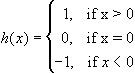#Interactive Real Analysis

Next | Previous | Glossary | Map

## 6.3. Discontinuous Functions

### Examples 6.3.4(b):

Prove that h(x) has a jump discontinuity at x = 0, and draw the graph of h(x)It is easy to see that the limit of h(x) as x approaches 0 from the left is -1, while the limit of h(x) as x approaches 0 from the right is +1. Hence, the left and right handed limits exist and are not equal, which makes x = 0 a jump discontinuity for this function.
Next | Previous | Glossary | Map